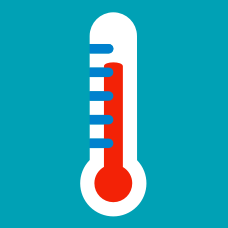Classical Mechanics

# Equilibrium

$$603600$$ gas particles are floating in a room. At equilibrium, walls are inserted into the room to divide the room into $$3$$ equal parts. About how many particles are there in each divided room?

We mix $$700 \text{ ml}$$ of a $$6 \%$$ solution of salt in water and $$700 \text{ ml}$$ of a $$4 \%$$ solution of sugar in water. At equilibrium, how much salt is in $$700 \text{ ml}$$ of the mixture solution?

Note

• $$X$$% indicates percent weight by volume. For example, 200 mL of a $$2$$% fructose solution contains 4 g fructose.

System $$A$$, consisting of $$22$$ particles, has total thermal energy $$E_1 = 88 \text{ J}.$$ System $$B$$, consisting of $$11$$ particles, has total thermal energy $$E_2 = 44 \text{ J}.$$ If system $$A$$ can exchange heat with system $$B$$, what is the thermal energy of each particle at equilibrium?

There are three boxes with red and white balls. The first box contains a total of $$200$$ balls: $$80$$ white balls and $$120$$ red balls. The second box contains $$400$$ balls, and we mix them with the $$200$$ balls in the third box. Then we re-divide those mixed balls between the second and third boxes, $$400$$ and $$200 ,$$ which are exactly the original numbers. Now, we conduct the same kind of mix-and-divide process with the third and first boxes, and find that there is no change in the ratio of red and white balls in the first box. How many red balls are in the second box?

Assumption

• Whenever we conduct the mix-and-divide process on any two boxes, the resulting ratio of white and red balls in each box is the same, which is true on average.

Particle $$A$$ with thermal energy $$E_1 = 26 \text{ J}$$ is placed in a box with particle $$B$$ with thermal energy $$E_2 = 8 \text{ J}.$$ At equilibrium, what is the average thermal energy of both particles?

×